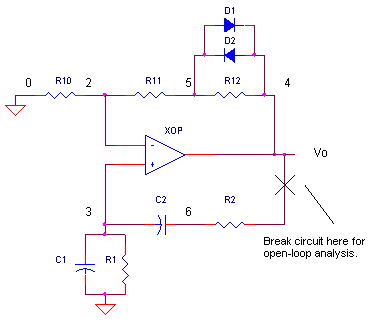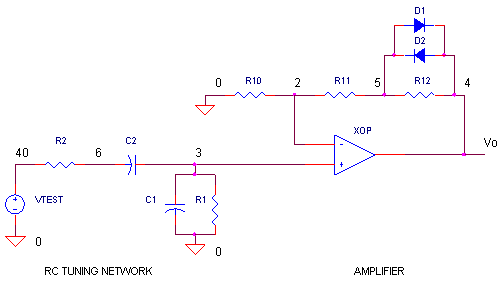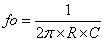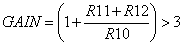eCircuit  Center

Wien Bridge Oscillator

CIRCUITThe opamp Wien-bridge oscillator provides a nice view into classic oscillator design using feedback analysis. Feedback analysis reveals if your circuit is stable (well behaved) or unstable (may oscillate). When designing amplifiers (especially high-speed ones), the trick is to avoid the conditions that make the circuit oscillate. When designing oscillators, you strive to achieve those conditions in a predictable way.

FEEDBACK ANALYSIS

Feedback analysis simply means opening the circuit and injecting an AC signal VTEST at one end of the circuit. Then, by looking at the magnitude (gain) and phase (time-shift) of signal as it travels around the opened loop, you can tell whether you’ve got an amplifier or an oscillator on your hands.

When wearing your oscillator design hat, the idea is to pick components that will make the open-loop analysis meet the conditions for oscillation at your chosen frequency: The conditions are as follows:

If the AC gain around the opened loop is 1 V/V and the total phase shift is -360 or 0 deg, the circuit will oscillate at that frequency.

OPENING THE LOOP

Open the loop of the Wien Bridge Oscillator at the op amp’s output. This is a good point because of the relatively low impedance of the output terminal. Likewise, VTEST also has a low output impedance. Therefore, opening the loop here does not significantly alter the circuit’s behavior.RC TUNING NETWORK

Two basic sections form the Wien-bridge oscillator: an RC tuning network and an amplifier. Both are necessary to achieve the conditions for oscillations. The RC network is characterized by a center frequencywhere R=R1=R2 and C=C1=C2. At the center frequency, two interesting things occur at V(3). First, the phase shift goes through 0 degrees. And second, the magnitude reaches a peak of 1/3 V/V.

CIRCUIT ANALYSIS   Run a SPICE simulation of the open-loop circuit OPWEIN_OL.CIR. Add trace VM(3) to see what the Magnitude looks like at the center frequency. For R1=R2=10k and C1=C2=16nF, the center frequency should be near 1 kHz. What does the phase look like? Add another plot window and then add trace VP(3) to see the Phase shift. The RC network does a nice job of meeting one of the conditions for oscillation: the phase is 0 degrees at the design frequency.

NON-INVERTING AMPLIFIER

The RC network falls short of the oscillation conditions in that the gain is only 1/3 V/V. How is the gain of 1V/V around the loop to be achieved? As you might have guessed, the non-inverting amplifier provides the needed gain. How much? A gain of 3 V/V makes the total gain 1/3 x 3 = 1 V/V.  Setting the correct op amp gain is critical. Not enough - oscillations will cease. Too much – oscillation amplitude will grow until the output saturates.

What’s needed is a mechanism to guarantee oscillations will start (GAIN > 3), yet, limit the gain (GAIN=3) at steady state. Enter our heros - D1, D2 and R12. The circuit adjusts its gain depending on the signal level. For small signals, the diodes do not conduct and the gain is set byFor larger signals, the voltage across R12 is big enough to make D1 and D2 conduct. The shunt resistance of the conducting diodes effectively reduces the R12 resistance, consequently, reducing the overall gain to GAIN=3.

CIRCUIT ANALYSIS   Run a simulation of OPWIEN_OL.CIR. View the AC output of the op amp VM(4). For R10=10k, R11=18k and R12=5k, the op amp gain is (1 + (18+5)/10) = 3.3 V/V. This should make the overall open-loop gain equal to 1/3 x 3.3 = 1.1 V/V. Does the peak at VM(4) reach this expected gain?

OSCILLATOR OPERATION

It’s time to close the loop and try out the Wien-Bridge Oscillator. Run a simulation of closed-loop circuit OPWIEN.CIR and plot the Transient Analysis at V(4). How much time does it take for the amplitude to stabilize?

HANDS-ON DESIGN   Design the circuit with a different oscillation frequency. Calculate the values for R and C. (Example: For fo = 10kHz, choose R1=R2=10k and calculate C1=C2=1/(2π x R1 x fo) = 1.6nF.) Test drive your oscillator. If there’s too little or too many sinewaves on the plot, adjust the total time of the Transient Analysis to another value like 5 ms by modifying the .TRAN statement to look like

.TRAN    0.05MS    5MS

If there’s no input signal to an oscillator, what starts the oscillations? Current source IS injects a pulse into the RC network to jump start the oscillations. In a real circuit, the large transient at power up will kick the circuit into action.

CIRCUIT INSIGHT    What happens if there’s not enough gain around the loop? Reduce R12 to 1k making the total loop gain less than 1. Run a simulation. The circuit rings briefly, but there’s not enough gain to sustain oscillations.

SPICE FILES

Download the file or copy this netlist into a text file with the *.cir extention.

OPWIEN_OL.CIR - OPAMP WIEN-BRIDGE OSC, OPEN-LOOP ANALYSIS
*
VTEST	40	0	AC	1
*
* RC TUNING
R2	40	6	10K
C2	6 	3	16NF
R1	3 	0	10K
C1	3 	0	16NF
* NON-INVERTING OPAMP
R10	0	2	10K
R11	2	5	18K
XOP	3 2	4	OPAMP1
* AMPLITUDE STABILIZATION
R12	5	4	5K
*D1	5	4	D1N914
*D2	4	5	D1N914
*
.model	D1N914	D(Is=0.1p Rs=16 CJO=2p Tt=12n Bv=100 Ibv=0.1p)
*
* OPAMP MACRO MODEL, SINGLE-POLE
* connections:      non-inverting input
*                   |   inverting input
*                   |   |   output
*                   |   |   |
.SUBCKT OPAMP1      1   2   6
* INPUT IMPEDANCE
RIN	1	2	10MEG
* DC GAIN (100K) AND POLE 1 (100HZ)
EGAIN	3 0	1 2	100K
RP1	3	4	1K
CP1	4	0	1.5915UF
* OUTPUT BUFFER AND RESISTANCE
EBUFFER	5 0	4 0	1
ROUT	5	6	10
.ENDS
*
*
* ANALYSIS
.AC	DEC 10	10 10MEG
* VIEW RESULTS
.PRINT	AC 	VM(3) VP(3)
.PLOT	AC 	VM(3) VP(3)
.PROBE
.END

Download the file or copy this netlist into a text file with the *.cir extention.

OPWIEN.CIR - OPAMP WIEN-BRIDGE OSCILLATOR
*
* CURRENT PULSE TO START OSCILLATIONS
IS	0	3	PWL(0US 0MA   10US 0.1MA   40US 0.1MA   50US 0MA   10MS 0MA)
*
* RC TUNING
R2	4	6	10K
C2	6 	3	16NF
R1	3 	0	10K
C1	3 	0	16NF
* NON-INVERTING OPAMP
R10	0	2	10K
R11	2	5	18K
XOP	3 2	4	OPAMP1
* AMPLITUDE STABILIZATION
R12	5	4	5K
D1	5	4	D1N914
D2	4	5	D1N914
*
.model	D1N914	D(Is=0.1p Rs=16 CJO=2p Tt=12n Bv=100 Ibv=0.1p)
*
* SINGLE-POLE OPERATIONAL AMPLIFIER MACRO-MODEL
* connections:      non-inverting input
*                   |   inverting input
*                   |   |   output
*                   |   |   |
.SUBCKT OPAMP1      1   2   6
* INPUT IMPEDANCE
RIN	1	2	10MEG
* DC GAIN (100K) AND POLE 1 (100HZ)
EGAIN	3 0	1 2	100K
RP1	3	4	1K
CP1	4	0	1.5915UF
* OUTPUT BUFFER AND RESISTANCE
EBUFFER	5 0	4 0	1
ROUT	5	6	10
.ENDS
*
* ANALYSIS
.TRAN 	0.05MS 10MS
*
* VIEW RESULTS
.PRINT	TRAN 	V(4)
.PLOT	TRAN 	V(4)
.PROBE
.END

top